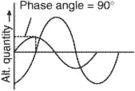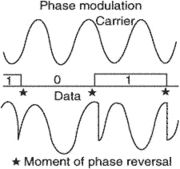# phase angle

Also found in: Dictionary, Thesaurus, Wikipedia.
Related to phase angle: phase difference

## phase angle

The angle between the lines connecting the Moon and Sun and the Moon and Earth or connecting a planet and the Sun and the planet and Earth (see illustration). At full or new Moon the phase angle is 0° or 180°, respectively. At first and last quarters it is 90°. For the superior planets phase angle is greatest at quadrature. For the inferior planets it is greatest (180°) at inferior conjunction and equals 90° at greatest elongation.

Collins Dictionary of Astronomy © Market House Books Ltd, 2006

## phase angle

[′fāz ‚aŋ·gəl]
(physics)
The difference between the phase of a sinusoidally varying quantity and the phase of a second quantity which varies sinusoidally at the same frequency. Also known as phase difference.
McGraw-Hill Dictionary of Scientific & Technical Terms, 6E, Copyright © 2003 by The McGraw-Hill Companies, Inc.

## phase anglei. An angle between the sight lines to the sun and the earth measured at a remote location (e.g., other celestial bodies).
ii. The number of electrical degrees between the time an AC (alternating current) voltage passes through zero moving in the positive direction and the time the current passes through zero going in the same direction.
iii. The phase difference between two sets of periodic phenomena expressed in an angular measure.
References in periodicals archive ?
The phase angle of the electrical impedance is a predictor of long-term survival in dialysis patients.
On that date Mercury's solar phase angle was 87[degrees] and its distance from the Sun was 0.45 au according to the website https://ssd.jpl.nasa.gov/horizons.cgi.
Figures 10-13 show the trajectories of the load phase angle versus time, the load voltage versus time, the generator phase angle versus time, and the generator rotor speed deviation versus time.
[DELTA]P > 0 means that the phase angle difference between [V.sub.ES] and [I.sub.SL] is 0[degrees] and the input power of smart load is decreased by ES, while [DELTA]P < 0 means that the phase angle difference between [V.sub.ES] and [I.sub.SL] is 180[degrees] and the input power of smart load is increased by ES.
At most of the operating frequencies beyond the resonant frequency range, the relative errors of the amplitude and phase angle measured by the PIA are small compared to those measured by the Agilent 4294a impedance analyzer.
Electrical impedance spectroscopy (EIS) is a multifrequency analysis for studying complex electrical impedance, Z([omega]), and its phase angle, 0(w), at different frequency points, [[omega].sub.i]([[omega].sub.i] : [[omega].sub.1], [[omega].sub.2], [[omega].sub.3], ..., [[omega].sub.n]).
For a viscoelastic material, the stress and strain will be out of phase by some quantity, the phase angle delta.
As far as the slamming force are concerned, cases with phase angle 0[degrees] have the biggest one, which is also the most dangerous situation for cylinder lowering through wave surface, mainly due to the biggest relative velocity between the cylinder and water particles, which can be observed from Figure 11.
This shift is measured geometrically as the angular transformation of the ratio of capacitance to resistance, or the phase angle (PA) [65-68].
The dynamic modulus and phase angle were collected during testing.
The latter impedance data were confirmed by the phase angle plot that better emphasizes these effects .
The double-frequency resonance is used to provide more wideband and flatter response for a single frequency band; and, simultaneously, the two resonances may be selected farther away from each other, resulting in two phase angle sections effectively usable for two different frequencies.

Site: Follow: Share:
Open / Close